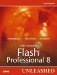# The ConvolutionFilter Class

### The ConvolutionFilter Class

The ConvolutionFilter class is designed to apply matrix convolution effects on objects.

It is important when using this class to import it first at the beginning of your script, like this:

` import flash.filters.ConvolutionFilter; `

To instantiate a new instance of the ConvolutionFilter class, use this code as a template:

`[View full width] var myFilter:ConvolutionFilter = new ConvolutionFilter(matrix, matrixY, matrix, divisor,bias, preserveAlpha, clamp, color, alpha); `

• matrixX The number of columns in the matrix. Default value of 0.

• matrixY The number of rows in the matrix. Default value of 0.

• matrix An Array with (matrixX x matrixY) elements for the transformation.

• divisor The divisor used for the matrix transformation. Default value of 1.

• bias The bias that is added to the matrix transformation result. Default value of 0.

• preserveAlpha A Boolean value that if true will make it so the convolution filter will not affect the alpha channel. Default value of true.

• clamp A Boolean value that if set to true will extend the bitmap along its edge and duplicate the existing colors; if false, color will be used.

• color A substitute color for pixels outside the bitmap.

• alpha The alpha value of color.

Example:

This example will create a small blue square using the drawing API and apply the convolution filter to it to make it a semitransparent gray square:

` import flash.filters.ConvolutionFilter; //create the movie clip to display the filter var rec_mc:MovieClip = this.createEmptyMovieClip("rec_mc", 1); //move the rectangle towards the center of the stage rec_mc._x = rec_mc._y = 200; //draw a squar inside the movie clip rec_mc.lineStyle(0,0x000000,0); rec_mc.beginFill(0x397dce, 100); rec_mc.lineTo(100,0); rec_mc.lineTo(100,100); rec_mc.lineTo(0,100); rec_mc.lineTo(0,0); rec_mc.endFill(); //create the matrix var matrix:Array = [0, 1, 0, 0, 1, 0, 0, 1, 0]; //create the filter var myFilter:ConvolutionFilter = new ConvolutionFilter(3, 3, matrix, 18, 1, false); //apply the filter rec_mc.filters = new Array(myFilter); `

Because the properties of this object match the parameters identically, they will be skipped.

#### Methods

##### clone

Availability: FP:8, AS:1.0

Generic Template: myFilter.clone()

Returns: ConvolutionFilter

An exact copy of the ConvolutionFilter will be returned.

Description:

This method will create a duplicate copy of the ConvolutionFilter it is called on.

Example:

This example will create a filter, clone it, and then walk through all the properties to see that they match:

` import flash.filters.ConvolutionFilter; //create the matrix var matrix:Array = [0, 1, 0, 0, 1, 0, 0, 1, 0]; //create the filter var myFilter:ConvolutionFilter = new ConvolutionFilter(3, 3, matrix, 18, 1, false); //create a copy var myNewFilter:ConvolutionFilter = myFilter.clone(); //walk through and display each property for(each in myNewFilter){     trace(each + ": " + myNewFilter[each]); } //output:clone: [type Function] //alpha: 0 //color: 0 //clamp: true //preserveAlpha: false //bias: 1 //divisor: 18 //matrix: 0,1,0,0,1,0,0,1,0 //matrixY: 3 //matrixX: 3 `Macromedia Flash Professional 8 Unleashed
ISBN: 0672327619
EAN: 2147483647
Year: 2005
Pages: 319